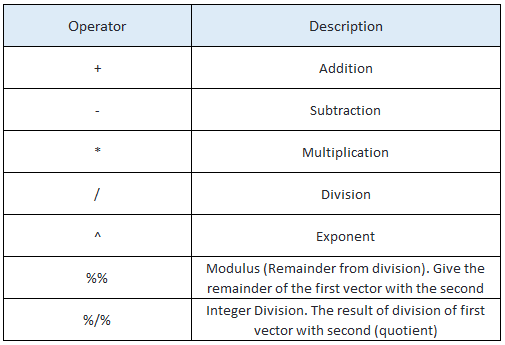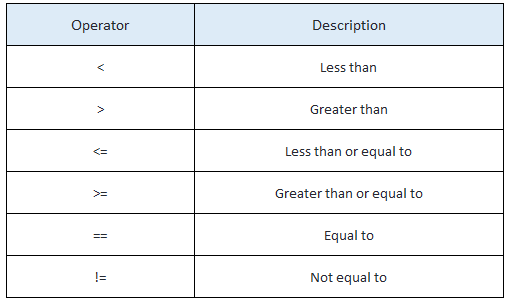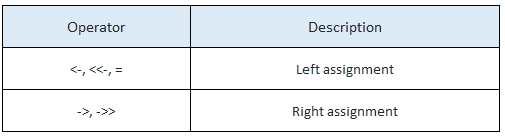### R – Operators

R language has so many  built-in operators to perform different arithmetic and logical operations. There are mainly 4 types of operators in R.

1. Arithmetic Operators
2. Relational Operators
3. Logical Operators
4. Assignment Operators
5. Mixed Operators

#### Arithmetic Operators in R:

Below table shows the arithmetic operators in R.x <- 35
y<-10

x+y
x-y
x*y
x/y
x%/%y
x%%y
x^y

Output:

> x+y
 45
> x-y
 25
> x*y
 350
> x/y
 3.5
> x%/%y
 3
> x%%y
 5
> x^y
 2.758547e+15

#### Relational Operators in R:

Below table shows the relational operators in R.x <- 35
y<-10

x<y
x>y
x>=35
x<=35
y==10
x!=y
y!=10

Output:

> x<y
 FALSE
> x>y
 TRUE
> x>=35
 TRUE
> x<=35
 TRUE
> y==10
 TRUE
> x!=y
 TRUE
> y!=10
 FALSE

#### Logical Operators in R

Below table shows the logical operators in R. Operators & and | perform element-wise operation producing result having length of the longer operand.But && and || examines only the first element of the operands resulting into a single length logical vector.See the below example properly, Zero is considered FALSE and non-zero numbers are taken as TRUE.a <- c(TRUE,TRUE,FALSE,0,6,7)
b <- c(FALSE,TRUE,FALSE,TRUE,TRUE,TRUE)
a&b
a&&b
a|b
a||b
!a
!b

Output:

> a&b
 FALSE TRUE FALSE FALSE TRUE TRUE
> a&&b
 FALSE
> a|b
 TRUE TRUE FALSE TRUE TRUE TRUE
> a||b
 TRUE
> !a
 FALSE FALSE TRUE TRUE FALSE FALSE
> !b
 TRUE FALSE TRUE FALSE FALSE FALSE

#### Assignment Operators in R:

Below table shows the assignment operators in R. They are used to assign values to variables.v1 <- 100
v2 <<-200
v3 = 300
v4<-c(1:10)

1000 -> v5
200->> v6
20:30->>v7
v1
v2
v3
v4
v5
v6
v7

Output:

> v1
 100
> v2
 200
> v3
 300
> v4
 1 2 3 4 5 6 7 8 9 10
> v5
 1000
> v6
 200
> v7
 20 21 22 23 24 25 26 27 28 29 30

#### Mixed Operators in R:

There are few other useful operators in R which are being used for specific purpose and not general mathematical or logical computation.x<- 1:10
y<- 8
z<-20
x
y %in% x
z %in% x

m<-matrix(1:9,3,3)
m%*%m
m*m

Output:

> x
 1 2 3 4 5 6 7 8 9 10
> y %in% x
 TRUE
> z %in% x
 FALSE
>
> m<-matrix(1:9,3,3)
> m%*%m
[,1] [,2] [,3]
[1,] 30 66 102
[2,] 36 81 126
[3,] 42 96 150
> m*m
[,1] [,2] [,3]
[1,] 1 16 49
[2,] 4 25 64
[3,] 9 36 81